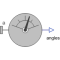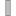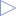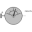# AbsoluteAngles

Measure absolute angles between frame connector and the world frame# Information

This information is part of the Modelica Standard Library maintained by the Modelica Association.

This model determines the 3 angles to rotate the world frame into frame_a along the axes defined by parameter sequence. For example, if sequence = {3,1,2} then the world frame is rotated around angles along the z-axis, afterwards it is rotated around angles along the x-axis, and finally it is rotated around angles along the y-axis and is then identical to frame_a. The 3 angles are returned in the range

```    -p <= angles[i] <= p
```

There are two solutions for "angles" in this range. Via parameter guessAngle1 (default = 0) the returned solution is selected such that |angles - guessAngle1| is minimal. The transformation matrix between the world frame and frame_a may be in a singular configuration with respect to "sequence", i.e., there is an infinite number of angle values leading to the same relative transformation matrix. In this case, the returned solution is selected by setting angles = guessAngle1. Then angles and angles can be uniquely determined in the above range.

The parameter sequence has the restriction that only values 1,2,3 can be used and that sequence ≠ sequence and sequence ≠ sequence. Often used values are:

```  sequence = {1,2,3}  // Cardan or Tait-Bryan angle sequence
= {3,1,3}  // Euler angle sequence
= {3,2,1}
```

# Parameters (2)

sequence Value: {1, 2, 3} Type: RotationSequence Description: Angles are returned to rotate world frame around axes sequence, sequence and finally sequence into frame_a Value: 0 Type: Angle (rad) Description: Select angles such that abs(angles - guessAngle1) is a minimum

# Connectors (2)

frame_a anglesType: Frame_a Description: Coordinate system a of which the absolute kinematic quantities are measuredType: RealOutput Description: Angles to rotate world frame into frame_a via 'sequence'

# Used in Components (1)AbsoluteSensor Modelica.Mechanics.MultiBody.Sensors Measure absolute kinematic quantities of frame connector